# Direct Junction Temperature Measurement of a DC Switching Power SupplyGet valuable resources straight to your inbox - sent out once per month

## Introduction

Designers often want to measure the junction temperature of a DC switching power supply. This is especially difficult in temperature chambers because a thermal camera is inaccurate and can be damaged under high environmental temperatures, and an external temperature sensor is difficult to fix onto small packages. This article will help readers understand a practical method to measure the junction temperature of an IC by demonstrating how to implement a direct readout temperature measuring method using the MOSFET body diode of a power good (PG) pin. This is a direct voltage readout method using the diode voltage vs. temperature relationship.

## Background

In many applications, it is important to measure the junction temperature under the maximum specified load and ambient temperature. Figure 1 shows the DC block diagram of MPS’s MPQ4572, which uses a PG body diode. This device will be used as the example for this article.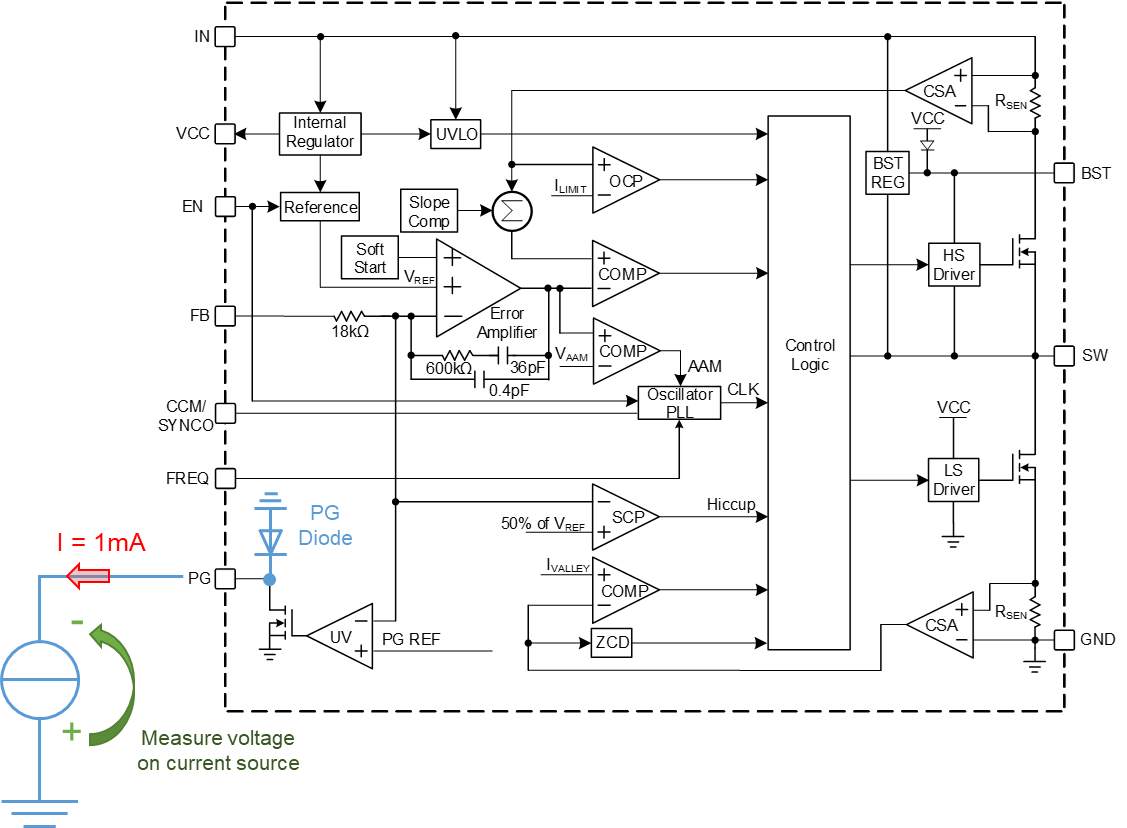Figure 1: PG N-Channel MOSFET Body Diode in the MPQ4572

The MPQ4572 is a fully integrated, fixed-frequency, synchronous step-down converter that can achieve up to 2A of continuous output current with peak current control. The device offers a 4.5V to 60V input voltage range to accommodate a variety of step-down applications. Apply a 1mA current source in the forward direction through the body diode (a part of the MOSFET) on the PG pin (see Figure 1).

The diode voltage vs. temperature curve can be measured on the EVQ4572-QB-00A evaluation board (see Figure 2). It can also be measured directly from a custom board. The diode curve characteristic depends on temperature, rather than on the PCB dimensions.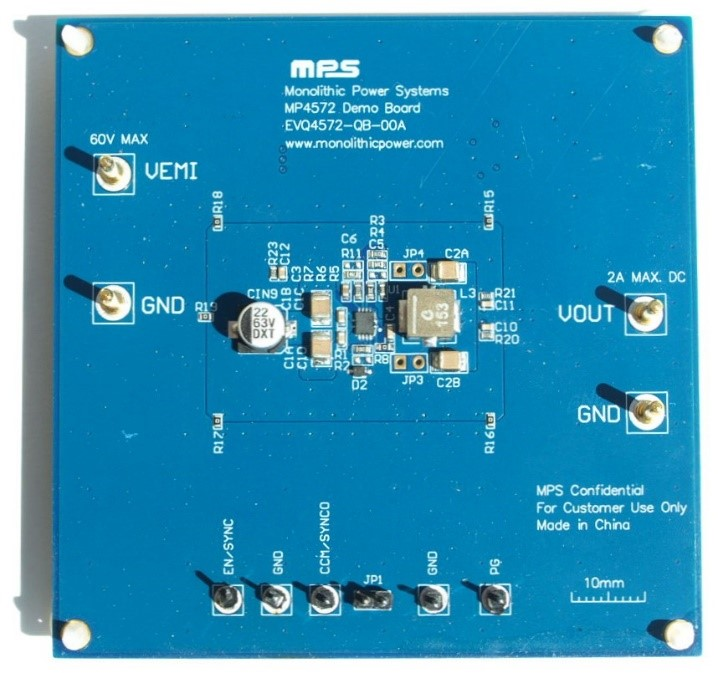Figure 2: EVQ4572-QB-00A 4-Layer Evaluation Board (8.9cmx8.9cm)

## Measuring the Junction Temperature with the Power Good (PG) Body Diode

The power good (PG) pin has an internal N-channel MOSFET with a body diode. To accurately measure the junction temperature, the forward diode voltage and temperature must be calibrated. Follow the steps below for calibration:

1. Disconnect any resistor, microcontroller, or part from the PG pin.
2. Glue a temperature sensor (e.g. a small PT1000, 4-wire) on top of the device package that is being tested. Another method is to solder a floating thermocouple close to the device being tested (it is recommended to solder this thermocouple to GND). Fixing temperature sensors to the package is a demanding task, so use the smallest possible sensors. The temperature sensor should not act as heatsink for the small package. Use thermal conductive glue to fix a PT1000 temperature sensor to the package, or use a thermocouple soldered directly to a part of the board that has EMC quiet potential (e.g. GND or VIN) (see Figure 3).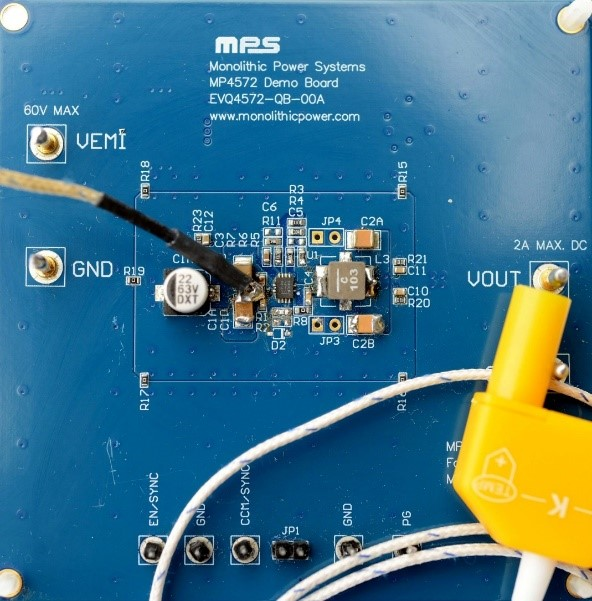Figure 3: Soldering a Themocouple to the PCB

1. Connect a precision multimeter with a built-in diode test function and a 1mA current source to the PG pin (see Figure 1 and 5). Smaller currents can be used, but the system must have the same current while it is calibrated and while measurements are taken.
2. Measure the forward diode voltage vs. junction temperature relationship in a climate chamber.
3. Measure the diode voltage when the device is powered by a supply voltage under the desired input voltage (VIN). Determine which VIN values have valid calibration because VIN can influence the efficiency, and can therefore influence the device temperature as well. Do not connect a load on the DC/DC converter output.
4. Take measurements with the evaluation board or on a custom PCB.
5. Turn the device off.
6. Start the climate chamber (for example, at 25°C), and ensure that the external temperature sensor shows a stable reading.
7. Turn the device on for a short period, and read the voltage on the multimeter. Without a load, the junction temperature should not rise significantly, because the power loss in the junction is low (only a few mW). If possible, use advanced asynchronous mode (AAM) due to its low quiescent current under small loads.
8. Turn the device off.
9. Set the climate chamber to the next selected temperature and let the PCB temperature settle for approximately 20 to 30 minutes, depending on the PCB’s specific heat capacity and size.
10. Turn the device on for a short period, and read the voltage on the multimeter.
11. Turn the device off again. Continue with the next selected temperature for the climate chamber.
12. Measure the forward diode voltage under the maximum desired load and maximum ambient temperature.

When measuring the PG forward voltage diode keep the following in mind:

• The slope of this calibrated voltage vs. junction temperature is almost linear. For the highest accuracy, use more points and a polynomial fit function. Check the calibration for repeatability.
• Devices of the same type have similar slopes, but often different offsets.
• Similar devices will typically have slightly different slopes.
• Side effects, such as a small change to VOUT, are possible. This should not be considered a failure, as the coupled currents within the junction can cause such effects.
• The main advantage of this measuring method is that the forward diode voltage can be used to calculate the junction temperature under any load.
• A temperature sensor is not necessary.
• Note that not every part can use the PG pin to measure the current; contact the part manufacturer for product guidance.

## Measured Calibration Curve

Figure 4 shows a first-order PG forward diode voltage vs. junction temperature graph, which has a linear fit function. The PG diode is driven by the external 1mA current source shown in Figure 1.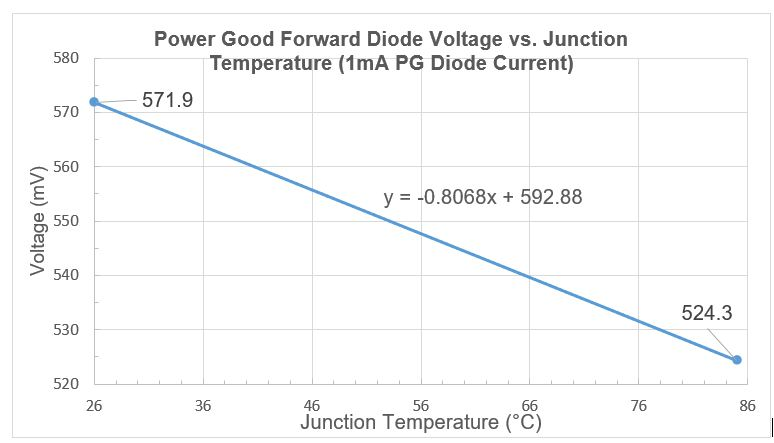Figure 4: Measured Calibration Curve on the EVQ4572-QB-00A

By measuring the diode voltage, the junction temperature can be calculated with Equation (1):

$$voltage(T_J)=-0.8068 \times T_J \times \frac{mV}{°C}+592.88mV$$

## Comparing a Body Diode Readout to a Thermal Camera

Table 1 shows a direct comparison between the readout of the junction temperature and a visual thermal camera. The ambient temperature is measured with a platinum resistance type PT1000 (1109Ω for 28.0°C).

Table 1: PG Forward Diode Temperature Readout vs. Thermal Camera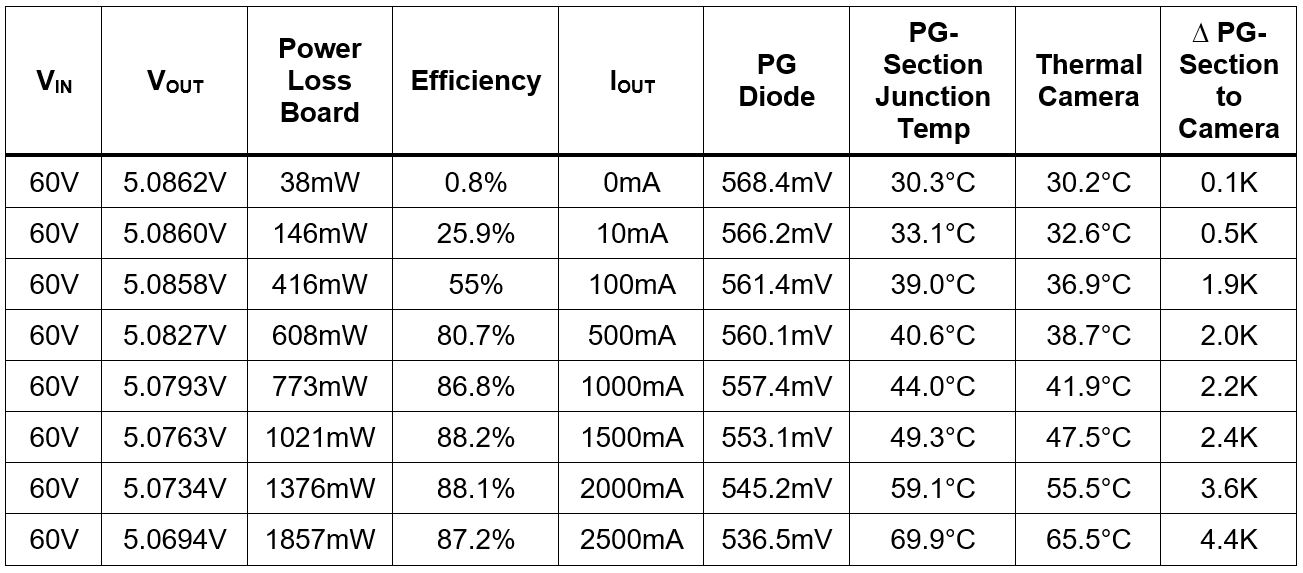Table 1 shows that the measured junction temperature is comparable to the thermal camera on the PG diode section of the package. The camera method shows lower temperatures, caused by thermal resistance of the mold compound between the junction and the package top surface. The camera is adjusted to a 0.95 emissivity, which is a good fit for the mold compound of the package. The junction temperature is not consistent between components (e.g. the PG section inside the die is colder than the MOSFET section). Figure 5 shows the PG diode section and MOSFET section.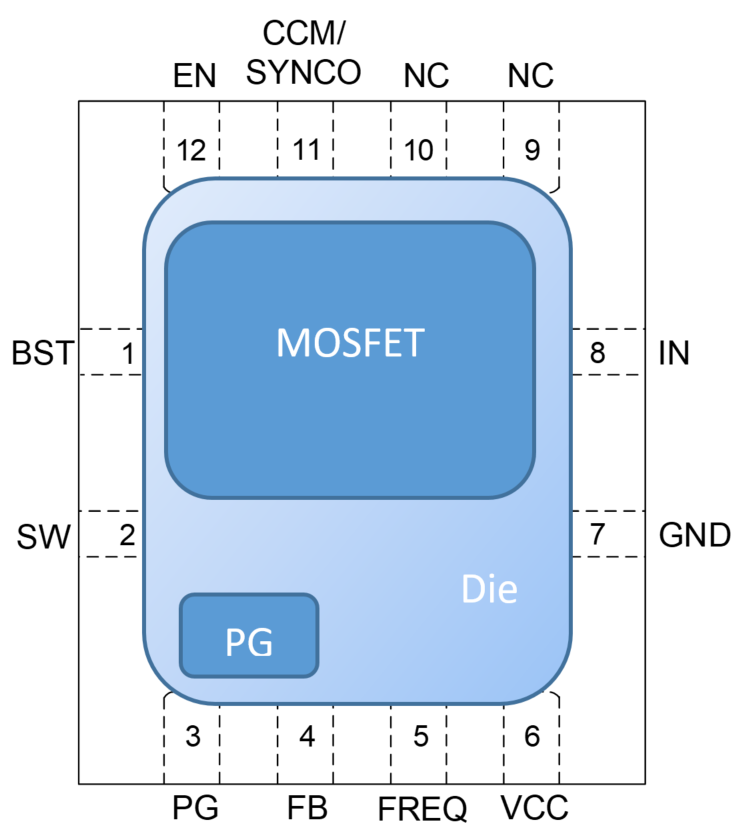Figure 5: MPQ4572 Package with MOSFET and PG Section

As shown in Figure 5 and Figure 10, the small signal section and the power MOSFET section are at different positions. The PG forward voltage diode measures the junction temperature at the PG position, so the diode temperature must be compared to the camera temperature at that position. Because the MOSFET has a higher temperature by a few degrees, that small offset must be added to the maximum junction temperature. Figures 6–13 show the camera measurements corresponding to Table 1. These measurements were all taken using the EVQ4572-QB-00A.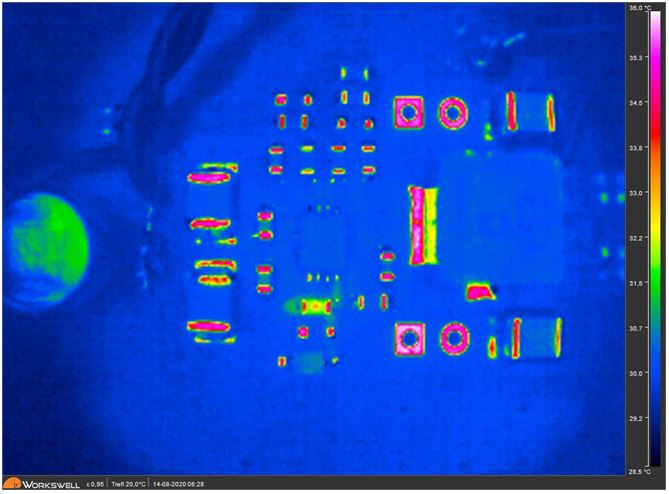Figure 6: Measurements when ILOAD = 0mA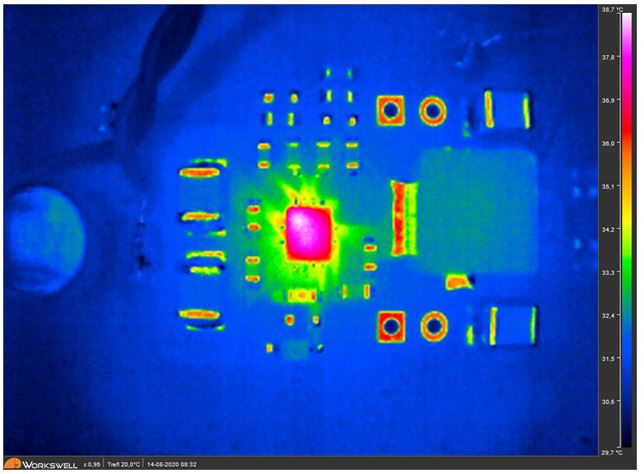Figure 8: Measurements when ILOAD = 100mA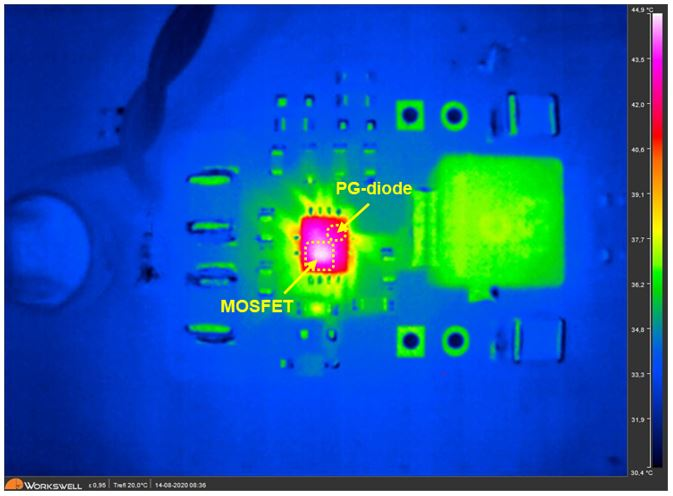Figure 10: Measurements when ILOAD = 1000mA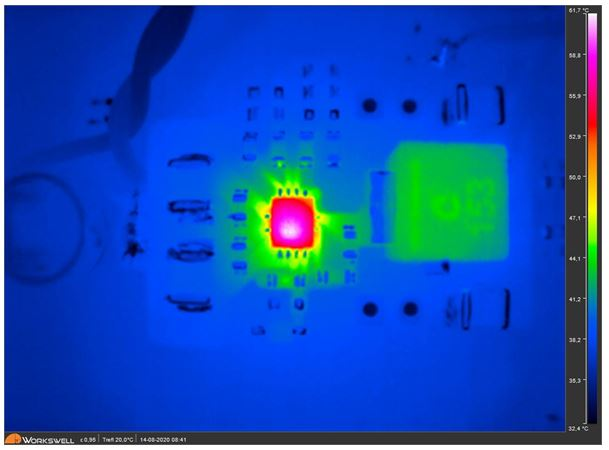Figure 12: Measurements when ILOAD = 2000mA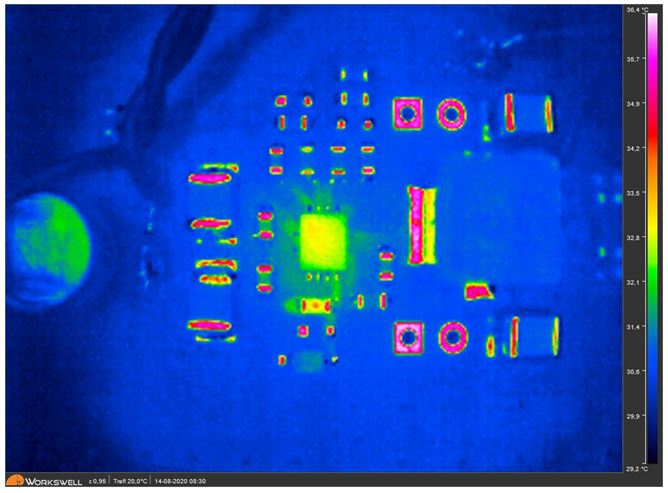Figure 7: Measurements when ILOAD = 10mA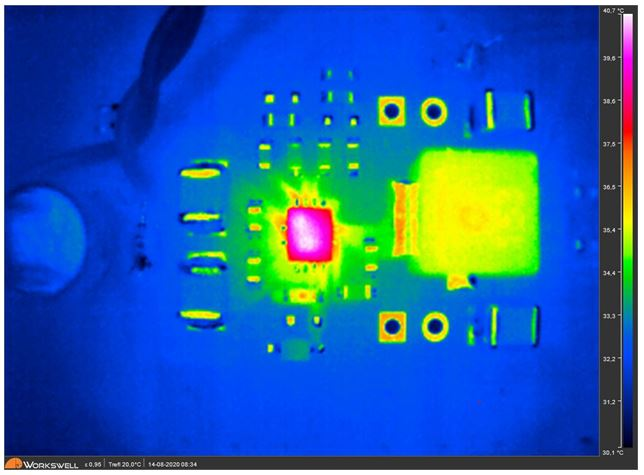Figure 9: Measurements when ILOAD = 500mA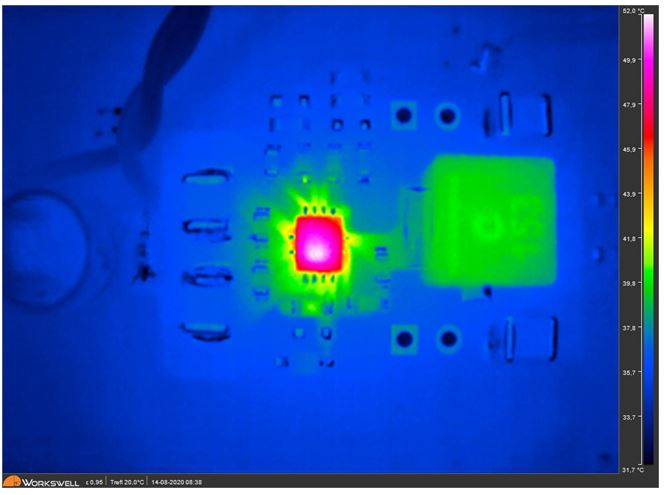Figure 11: Measurements when ILOAD = 1500mA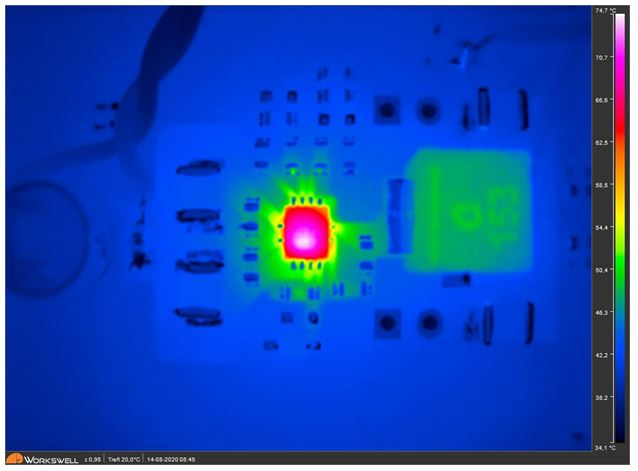Figure 13: Measurements when ILOAD = 2500mA (1)

Note:

1) A continuous 2.5A current is not recommended.

## Conclusion

Knowing a system’s junction temperature is an important and basic requirement for many safety and FMEA considerations.

This direct temperature readout method simplifies the process for design engineers when testing a custom PCB in a temperature chamber, where a thermal camera cannot be utilized. This helpful method achieves fast, reliable, and accurate junction temperature data without the complicated and often time-intensive process such as fixing temperature sensors on a device package.

### Did you find this interesting? Get valuable resources straight to your inbox - sent out once per month!

Get technical support